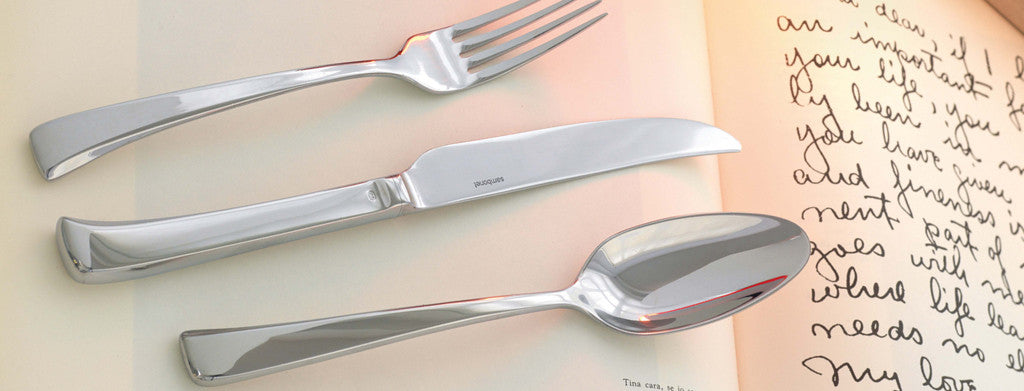Shopping Cart

### Refine

View all

#### Price# Cutlery£1.71 each (ex VAT)£1.98 each (ex VAT)£1.43 each (ex VAT)£1.71 each (ex VAT)£0.31 each (ex VAT)£0.55 each (ex VAT)£0.88 each (ex VAT)£0.34 each (ex VAT)£0.31 each (ex VAT)£1.04 each (ex VAT)£0.55 each (ex VAT)£0.53 each (ex VAT)£0.52 each (ex VAT)£0.97 each (ex VAT)£0.52 each (ex VAT)£1.43 each (ex VAT)£0.76 each (ex VAT)£0.59 each (ex VAT)£0.85 each (ex VAT)£1.30 each (ex VAT)£0.55 each (ex VAT)£1.04 each (ex VAT)£0.55 each (ex VAT)£1.04 each (ex VAT)£0.53 each (ex VAT)£0.53 each (ex VAT)£0.97 each (ex VAT)£0.53 each (ex VAT)£0.34 each (ex VAT)£0.34 each (ex VAT)£0.55 each (ex VAT)£1.04 each (ex VAT)£0.46 each (ex VAT)£0.87 each (ex VAT)£1.43 each (ex VAT)£1.61 each (ex VAT)£1.43 each (ex VAT)£1.21 each (ex VAT)£1.21 each (ex VAT)£1.21 each (ex VAT)£0.73 each (ex VAT)£0.83 each (ex VAT)£1.03 each (ex VAT)£1.43 each (ex VAT)£1.82 each (ex VAT)£1.43 each (ex VAT)£0.86 each (ex VAT)£1.31 each (ex VAT)